• 1、掌握常规双边带调幅与解调的原理及实现方法。 2、掌握二极管包络检波原理。 3、掌握调幅信号的频谱特性。（选做） 4、了解常规双边带调幅与解调的优缺点。 常规双边带调幅与解调实验数据
• 武汉理工大学信号分析处理课程设计《基于Mablab的抑制载波双边带带幅度调制与解调分析》Matlab
• 探 探2007级学生数字通探 % 信原理课程设计 % TOC \o "1-5" \h \z 探 数字通信原理课程设计报告书 基于MATLAB的抑制载波双边带调 课题名称 幅和解调的实现设计 姓 名 学 号 院系部 物理与电信工程系 专 业 通信工程 ...
• 此matlab代码，给出了现代通信原理中的常规双边带调幅(AM)的matlab仿真程序，给出了调制与解调的过程，并分析了在不同信噪比条件下的解调性能。
• 用滤波法实现单边带调制，是分双边带信号形成和无用边带抑制两步完成的。双边带信号由平衡调制器形成。由于调制器的平衡作用，载频电平被抑制到很低。对无用边带的抑制，是由紧跟在平衡调制器后面的边带滤波器完成的...
调制和解调单边带调制和解调的方法有多种，其中最常用的是滤波法。用滤波法实现单边带调制，是分双边带信号形成和无用边带抑制两步完成的。双边带信号由平衡调制器形成。由于调制器的平衡作用，载频电平被抑制到很低。对无用边带的抑制，是由紧跟在平衡调制器后面的边带滤波器完成的。边带滤波器是一带通滤波器，若下边带为无用边带，则恰当地选择其中心频率和通带宽度，让上边带信号通过而抑制下边带。当需要形成多路独立边带信号时，就需要有相应数目的单边带信号产生器，它们具有不同的载频和不同中心频率的边带滤波器。然后把这些占有不同频段的单边带信号线性相加，便可得到多路独立边带信号。 　　单边带信号的解调，除了载频全发送的兼容单边带和残留单边带可以用包络检波外，其他各类单边带的解调只能用单边带产生的相反过程来完成（图3b），即仍用平衡调制器完成单边带信号频谱向基带的平移，并通过紧跟在调制器后面的低通滤波器，提取有用的基带信号，抑制无用的边带信号。 　　用滤波法产生和解调单边带信号，通常都在低于工作频率的低载频上进行。因此，在单边带产生器后和单边带解调器前有一个频率搬移部分，把单边带信号频谱从低载频搬移到工作频率，或相反。除滤波法外，还有相位补偿法和合成法可以在工作频率上直接产生单边带信号，但由于性能都不如滤波法，所以很少采用。

本设计的具体程序如下：

（1）利用matlab绘制已知信号f(t)

t=-2:0.001:2  %%信号f(t)

y1=sinc(t*200)

subplot(2,3,1),plot(t,y1) %画出原始信号

title('已知信号')

xlabel('时间：s')

ylabel('幅度')

grid

xlim([-0.1,0.1])

（2）利用matlab绘制已知信号f(t)的频谱

fs=3000 %%信号频谱

t1=-2:0.0001:2

y11=sinc(t1*200)

yk=fft(y11,50000)       %对信号做傅立叶变换

yw=2*pi/40000*abs(fftshift(yk))   %频谱搬移

fw=[-25000:24999]/50000*fs

subplot(2,3,2),plot(fw,yw)

title('已知信号的频谱')

xlabel('频率：hz')

ylabel('幅度')

grid

xlim([-30,30])

（3）利用matlab绘制载波信号

y3=cos(2*pi*200*t) %%载波信号

subplot(2,3,3),plot(t,y3)

title('载波信号')

xlabel('时间：s')

ylabel('幅度')

grid

xlim([-0.1,0.1])

（4）利用matlab绘制已调信号

y4=sinc(t*200).*cos(2*pi*200*t) %%已调信号

subplot(2,3,4),plot(t,y4,'r-')

title('已调信号')

xlabel('时间：s')

ylabel('幅度')

grid

xlim([-0.05,0.05])

(5) 利用matlab绘制已调信号的频谱

fs1=1000  %已调信号频谱

yk=fft(y4,5000)         %对信号做傅立叶变换

yw=2*pi/4000*abs(fftshift(yk))    %频谱搬移

fw=[-2500:2499]/5000*fs1

subplot(2,3,5),plot(fw,yw,'r-')

title('已调信号的频谱')

xlabel('频率：hz')

ylabel('幅度')

grid

xlim([-400,400])

（6）利用matlab绘制DSB-SC调制信号的功率谱密度

[c,lags]=xcorr(y4,200) %%DSB信号自相关函数

Figure             %200表示自相关函数时间т

subplot(211)

plot(lags/fs,c)

title('DSB信号自相关函数')

xlabel('t')

ylabel('Rxx(t)')

Grid

SDSBp=fft(c,5000) %%DSB功率谱

fw=[-2500:2499]/5000*fs1

yw=2*pi/4000*abs(fftshift(SDSBp))%频谱搬移

subplot(212)

plot(fw,yw)

title('DSB信号功率谱')

xlabel('w')

ylabel('Rxx(t)')

grid

（7）利用matlab绘制相干解调后的信号波形

y7=y4.*y3  %%解调信号

figure

subplot(211)

plot(t,y7)

title('解调信号')

xlabel('时间：s')

ylabel('幅度')

grid

xlim([-0.1,0.1])

Rp=0.1; %%滤波后的f(t)信号

Rs=80;                      %信号衰减幅度

Wp=40/100;                  %通带截止频率

Ws=45/100;                  %阻带截止频率,100为载波频率的一半

[n,Wn]=ellipord(Wp,Ws,Rp,Rs);   %阶数n

[b,a]=ellip(n,Rp,Rs,Wn);        %传递函数分子分母b,a

Xl=5*filter(b,a,y7);

figure;

subplot(211);

plot(t,Xl);

title('滤波后的f(t)信号');

xlabel('时间单位:s');

ylabel('幅度');

grid;

xlim([-0.1,0.1])


展开全文• DSB双边带信号调制，加噪声后解调，以及各个时刻的功率谱，频谱，自相关，时域波形。
• o(t)}mo​(t) - 时域波形 图表1 表示移频滤波解调后的调制信号 mo(t)=sd(t)∗h(t)\color{Brown}{m_o(t) = s_d(t) * h(t)}mo​(t)=sd​(t)∗h(t) 图表2 表示原基带信号 m(t)=cos⁡(20πt)\color{Brown}{m(t) = \cos...

0. 作业要求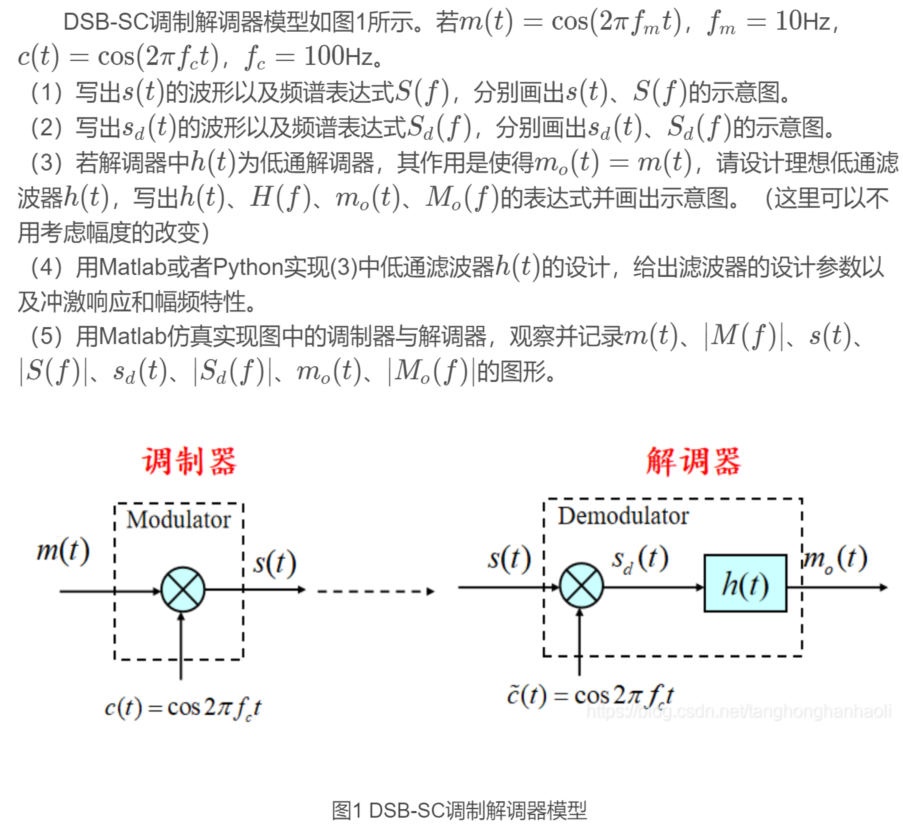1. $\color{Brown}{m(t), c(t), s(t)}$ 与 $\color{Brown}{M(f), C(f), S(f)}$ 的表达式与波形图
1.1 表达式
\begin{alignedat}{2} s(t) & = m(t) c(t) = \cos(20 \pi t)\cos(200 \pi t) \\ S(f) & = \frac{1}{4} \bigg [\delta(f - 110) + \delta(f - 90) + \delta(f + 90) + \delta(f + 110) \bigg ] \end{alignedat}
1.2 波形图

$\color{Brown}{m(t), c(t), s(t)}$ - 时域波形图表1 表示基带信号 $\color{Brown}{m(t) = \cos(20 \pi t)}$, 频率 $f_m = 10Hz$
图表2 表示载波信号 $\color{Brown}{c(t) = \cos(200 \pi t)}$, 频率 $f_c = 100Hz$
图表3 表示调制信号 $\color{Brown}{s(t) = m(t) c(t) = \cos(20 \pi t)\cos(200 \pi t)}$

$\color{Brown}{M(f)}$ - 频域波形图表1 表示信号 m(t) 的幅度频谱 |M(f)|
图表2 表示信号 m(t) 的相位频谱 θᴍ(f)
图表3 表示信号 m(t) 频率区间为 0~250Hz 的幅度频谱 |M(f)|
图表4 表示信号 m(t) 频率区间为 0~250Hz 的相位频谱 θᴍ(f)

$\color{Brown}{C(f)}$ - 频域波形图表1 表示信号 c(t) 的幅度频谱 |C(f)|
图表2 表示信号 c(t) 的相位频谱 θᴄ(f)
图表3 表示信号 c(t) 频率区间为 0~250Hz 的幅度频谱 |C(f)|
图表4 表示信号 c(t) 频率区间为 0~250Hz 的相位频谱 θᴄ(f)

$\color{Brown}{S(f)}$ - 频域波形图表1 表示信号 s(t) 的幅度频谱 |S(f)|
图表2 表示信号 s(t) 的相位频谱 θs(f)
图表3 表示信号 s(t) 频率区间为 0~250Hz 的幅度频谱 |S(f)|
图表4 表示信号 s(t) 频率区间为 0~250Hz 的相位频谱 θs(f)

2. $\color{Brown}{s_d(t)}$ 与 $\color{Brown}{S_d(f)}$ 的表达式与波形图
2.1 表达式
\begin{alignedat}{2} & \begin{aligned} s_d(t) & = s(t) \tilde{c}(t) \\ & = s(t) \cos(200 \pi t) \\ & = m(t) \cos^2(200 \pi t) \\ & = m(t) \frac{\cos(400 \pi t + 1)}{2} \\ & = \frac{1}{2} m(t) + \frac{1}{2} m(t) \cos(400 \pi t) \\ & = \frac{1}{2} \cos(20 \pi t) + \frac{1}{2} \cos(20 \pi t) \cos(400 \pi t) \\ \end{aligned} \\ & S_d(f) = \frac{1}{4} \bigg [\delta(f - 10) + \delta(f + 10) \bigg ] + \frac{1}{8} \bigg [\delta(f - 210) + \delta(f - 190) + \delta(f + 190) + \delta(f + 210) \bigg ] \end{alignedat}
2.2 波形图

$\color{Brown}{s(t) \ \tilde{c}(t) \ s_d(t)}$ - 时域波形图表1 表示调制信号 $\color{Brown}{s(t) = m(t) c(t) = \cos(20 \pi t)\cos(200 \pi t)}$
图表2 表示移频信号 $\color{Brown}{\tilde{c}(t) = \cos(200 \pi t)}$, 频率 $f_c = 100Hz$
图表2 表示移频后的调制信号 $\color{Brown}{s_d(t) = \frac{1}{2} \cos(20 \pi t) + \frac{1}{2} \cos(20 \pi t) \cos(400 \pi t)}$

$\color{Brown}{S_d(f)}$ - 频域波形图表1 表示信号 sᴅ(t) 的幅度频谱 |Sᴅ(f)|
图表2 表示信号 sᴅ(t) 的相位频谱 θs(f)
图表3 表示信号 sᴅ(t) 频率区间为 0~100Hz 的幅度频谱 |Sᴅ(f)|
图表4 表示信号 sᴅ(t) 频率区间为 0~100Hz 的相位频谱 θsᴅ(f)

3. $\color{Brown}{h(t)}$, $\color{Brown}{H(f)}$ 与 $\color{Brown}{m_o(t)}$, $\color{Brown}{M_o(f)}$ 的表达式与波形图
3.1 表达式
\begin{alignedat} \ h(t) = 4f_HSa(2 \pi f_H t) & \overset{\mathcal{F}}{\longleftrightarrow} H(f) = 2Rect(\frac{f}{2f_H}) \\ m_o(t) = s_d(t) * h(t) = \cos(20 \pi t) & \overset{\mathcal{F}}{\longleftrightarrow} M_o(f) = S_d(t)H(f) = \frac{1}{2} \bigg [\delta(f - 10) + \delta(f + 10) \bigg ] \\ \end{alignedat}
3.2 波形图

$\color{Brown}{h(t)}$ - 时域波形图表1 表示抽样函数 $\color{Brown}{h(t) = 4f_HSa(2 \pi f_H t)}$

$\color{Brown}{H(f)}$ - 频域波形图表1 表示抽样函数 h(t) 的幅度频谱 |H(f)|
图表2 表示抽样函数 h(t) 的相位频谱 θʜ(f)
图表3 表示抽样函数 h(t) 频率区间为 0~250Hz 的幅度频谱 |H(f)|
图表4 表示抽样函数 h(t) 频率区间为 0~250Hz 的相位频谱 θʜ(f)

$\color{Brown}{m_o(t)}$ - 时域波形图表1 表示移频滤波解调后的调制信号 $\color{Brown}{m_o(t) = s_d(t) * h(t)}$
图表2 表示原基带信号 $\color{Brown}{m(t) = \cos(20 \pi t)}$
图表2 表示去除滤波器产生的延迟后的解调信号 $\color{Brown}{m_o(t) = \cos(20 \pi t)}$

$\color{Brown}{M_o(f)}$ - 频域波形图表1 表示信号 mᴏ(t) 的幅度频谱 |Mᴏ(f)|
图表2 表示信号 mᴏ(t) 的相位频谱 θᴍ(f)
图表3 表示信号 mᴏ(t) 频率区间为 0~250Hz 的幅度频谱 |Mᴏ(f)|
图表4 表示信号 mᴏ(t) 频率区间为 0~250Hz 的相位频谱 θᴍ(f)

4. 其他
4.1 参考文章

 MATLAB通信仿真实例1：无噪声信道下DSB-SC调制解调器

4.2 仿真代码
# DSB-SC.py
# %% 配置导入
from scipy import signal
from scipy import fft
import scipy.constants as const
import matplotlib.pyplot as plt
import numpy as np

# %% 参数设置
time_begin = 0  # 起始时间
time_end = 1  # 终止时间
points = 1001  # 栅网采样点数
T = (time_end - time_begin) / (points - 1)  # 栅网采样间距
frequency = [10, 100]  # 频率
amplitude = [1, 1]  # 振幅
phase = [0, 0]  # 相位(cos(t)相位为0)
zoom = 4  # 频谱缩放倍数
tick_max = max(amplitude) * 1.5  # 波形纵轴刻度最小值
tick_min = -tick_max  # 波形纵轴刻度最大值

# %% 生成时域波形
t = np.linspace(time_begin, time_end, points)  # 生成时域栅网
waves = []
for i in range(len(frequency)):
waves.append(
signal.chirp(
t,
f0=frequency[i],
t1=time_end,
f1=frequency[i],
phi=phase[i],
method='linear'
) * amplitude[i]
)
s = None
for wave in waves:
if s is None:
s = wave.copy()
else:
s *= wave
s_d = waves * waves * waves

# %% 获得频域波形
f = np.linspace(0, 1 / T, points)  # 生成频域栅网
# 输入信号 M(f)
M = fft.fft(waves)  # 快速傅里叶变换
M_nor = M / points  # 归一化
M_mod = np.abs(M_nor)  # 获取幅度频谱
M_pha = np.angle(M)  # 获取相位频谱
# 移频信号 C(f)
C = fft.fft(waves)  # 快速傅里叶变换
C_nor = C / points  # 归一化
C_mod = np.abs(C_nor)  # 获取幅度频谱
C_pha = np.angle(C)  # 获取相位频谱
# 第一次移频 -> S(f)
S = fft.fft(s)  # 快速傅里叶变换
S_nor = S / points  # 归一化
S_mod = np.abs(S_nor)  # 获取幅度频谱
S_pha = np.angle(S)  # 获取相位频谱
# 第二次移频 -> S_d(f)
S_d = fft.fft(s_d)
S_d_nor = S_d / points  # 归一化
S_d_mod = np.abs(S_d_nor)  # 获取幅度频谱
S_d_pha = np.angle(S_d)  # 获取相位频谱

# %% 滤波器参数
f_H = 20  # 上限截止频率
time_begin_h = 0
time_end_h = 0.5
points_h = 501
T_h = (time_end_h - time_begin_h) / (points_h - 1)
frequency_h = f_H
amplitude = 4 * f_H
phase_h = -90
zoom_h = 10
tick_max_h = 4 * f_H
tick_min_h = -tick_max_h

# %% 生成抽样信号
t_h = np.linspace(time_begin_h, time_end_h, points_h)
h = amplitude * signal.chirp(
t_h,
f0=frequency_h,
t1=time_end_h,
f1=frequency_h,
phi=phase_h,
method='linear'
)  # 获得正弦函数
# 获得半个抽样信号
h = 4 * f_H
h[1:] /= (2 * const.pi * f_H * t_h[1:])
# 对称延拓抽样信号
t_h = np.hstack((-t_h[-1:0:-1], t_h))
h = np.hstack((h[-1:0:-1], h))

# %% 获得滤波器频域波形
f_h = np.linspace(0, 1 / T, points)  # 生成频域栅网
H = fft.fft(h)  # 快速傅里叶变换
H_nor = H / points  # 归一化
H_mod = np.abs(H_nor)  # 获取幅度频谱
H_pha = np.angle(H)  # 获取相位频谱

# %% 进行滤波, 获得 m_o(t) 的时域波形
m_o_original = signal.convolve(s_d, h) / points  # s_d(t) 与 h(t) 卷积
t_m_original = np.linspace(
time_begin, T * (len(m_o_original) - 1), len(m_o_original))  # m_o(t) 信号的栅网
m_o = m_o_original[points//2:points + points//2]  # 将通过滤波器之后产生的延迟截断

# %% 获得 m_o(t) 的频域波形
M_o = fft.fft(m_o)
M_o_nor = M_o / points  # 归一化
M_o_mod = np.abs(M_o_nor)  # 获取幅度频谱
M_o_pha = np.angle(M_o)  # 获取相位频谱

# %% 波形绘制函数
def drawWave(
figure_i,
row_i,
column_j,
label_x,
axis_xs,
axis_ys,
formats,
labels,
label_locs,
limit_ys,
linewidths):
"""
param:
row_i: 图表的行数
column_j: 图表的列数
label_x: 横轴标签
axis_xs: 横轴
axis_ys: 纵轴
formats: 曲线格式(颜色 风格 标记)
颜色:
'b' 蓝色(blue)(默认)
'g' 绿色(green)
'r' 红色(red)
'y' 黄色(yellow)
'k' 黑色(black)
'w' 白色(white)
'c' 青绿色(cyan)
'm' 洋红色(magenta)
'#xxxxxx'   RGB颜色
'0.xxx' 灰度
风格:
'-' 实线(默认)
':' 虚线
' ' 无线条
'--'    破折线
'-.'    点划线
标记:
'.' 点(默认)
',' 极小点
'o' 实心圆
'^' 正三角
'v' 倒三角
'>' 右三角
'<' 左三角
'1' 下花三角
'2' 上花三角
'3' 左花三角
'4' 右花三角
's' 实心方形(square)
'p' 实心五边形(pentagon)
'*' 星形
'h' 竖六边形(hexagon)
'H' 横六边形
'+' 十字
'x' 叉形
'D' 菱形(diamond)
'd' 瘦菱形
'|' 垂直线
labels: 曲线标签内容
label_locs: 曲线标签位置
0 "best 最佳位置(默认)
1 "upper right" 右上
2 "upper left" 左上
3 "lower right" 右下
4 "lower left" 左下
limit_ys: 坐标轴下限与上限
linewidths: 线宽
"""

fig = plt.figure(figure_i)
chart_formate = row_i * 100 + column_j * 10
for i in range(row_i * column_j):
ax = fig.add_subplot(chart_formate + 1 + i)
ax.plot(
axis_xs[i],
axis_ys[i],
formats[i],
label=labels[i],
linewidth=linewidths[i]
)  # 横轴, 纵轴, 标签
ax.legend(loc=label_locs[i])  # 标签位置
if limit_ys[i] is not None:
ax.set_ylim(limit_ys[i])  # 纵轴刻度范围
ax.grid()  # 显示网格线
ax.set_xlabel(label_x)

# %% 绘制 m(t) c(t) s(t) 时域波形
drawWave(
figure_i=1,
row_i=3,
column_j=1,
label_x=r"t / s",
axis_xs=[t] * 3,
axis_ys=waves + [s],
formats=['b-,', 'g-,', 'r-,'],
labels=[r"m(t) = cos(20πt)",
r"c(t) = cos(200πt)",
r"s(t) = m(t)c(t)"],
label_locs=[r"upper right"] * 3,
limit_ys=[(tick_min, tick_max)] * 3,
linewidths= * 3
)

# %% 绘制 m(t) 频域波形
drawWave(
figure_i=2,
row_i=4,
column_j=1,
label_x=r"f / Hz",
axis_xs=[np.hstack((-f[-1:0:-1], f))] * 2 + [f[0:points//zoom]] * 2,
axis_ys=[np.hstack((M_mod[-1:0:-1], M_mod)),
np.hstack((-M_pha[-1:0:-1], M_pha)),
M_mod[0: points // zoom],
M_pha[0: points // zoom]],
formats=['b-,', 'g-,'] * 2,
labels=[r"|M(f)|", r"θᴍ(f)"] * 2,
label_locs=[r"upper right"] * 4,
limit_ys=[(0, 0.6), None] * 2,
linewidths= * 4
)

# %% 绘制 c(t) 频域波形
drawWave(
figure_i=3,
row_i=4,
column_j=1,
label_x=r"f / Hz",
axis_xs=[np.hstack((-f[-1:0:-1], f))] * 2 + [f[0:points//zoom]] * 2,
axis_ys=[np.hstack((C_mod[-1:0:-1], C_mod)),
np.hstack((-C_pha[-1:0:-1], C_pha)),
C_mod[0: points // zoom],
C_pha[0: points // zoom]],
formats=['b-,', 'g-,'] * 2,
labels=[r"|C(f)|", r"θᴄ(f)"] * 2,
label_locs=[r"upper right"] * 4,
limit_ys=[(0, 0.6), None] * 2,
linewidths= * 4
)

# %% 绘制 s(t) 频域波形
drawWave(
figure_i=4,
row_i=4,
column_j=1,
label_x=r"f / Hz",
axis_xs=[np.hstack((-f[-1:0:-1], f))] * 2 + [f[0:points//zoom]] * 2,
axis_ys=[np.hstack((S_mod[-1:0:-1], S_mod)),
np.hstack((-S_pha[-1:0:-1], S_pha)),
S_mod[0: points // zoom],
S_pha[0: points // zoom]],
formats=['b-,', 'g-,'] * 2,
labels=[r"|S(f)|", r"θs(f)"] * 2,
label_locs=[r"upper right"] * 4,
limit_ys=[(0, 0.3), None] * 2,
linewidths= * 4
)

# %% 绘制 s(t) c(t) s_d(t) 时域波形
drawWave(
figure_i=5,
row_i=3,
column_j=1,
label_x=r"t / s",
axis_xs=[t] * 3,
axis_ys=[s, waves, s_d],
formats=['b-,', 'g-,', 'r-,'],
labels=[r"s(t) = m(t)c(t)",
r"c(t) = cos(200πt)",
r"s_d(t) = s(t)c(t)"],
label_locs=[r"upper right"] * 3,
limit_ys=[(tick_min, tick_max)] * 3,
linewidths= * 3
)

# %% 绘制 s_d(t) 频域波形
drawWave(
figure_i=6,
row_i=4,
column_j=1,
label_x=r"f / Hz",
axis_xs=[np.hstack((-f[-1:0:-1], f))] * 2 + [f[0:points//zoom]] * 2,
axis_ys=[np.hstack((S_d_mod[-1:0:-1], S_d_mod)),
np.hstack((-S_d_pha[-1:0:-1], S_d_pha)),
S_d_mod[0: points // zoom],
S_d_pha[0: points // zoom]],
formats=['b-,', 'g-,'] * 2,
labels=[r"|Sᴅ(f)|", r"θsᴅ(f)"] * 2,
label_locs=[r"upper right"] * 4,
limit_ys=[(0, 0.3), None] * 2,
linewidths= * 4
)

# %% 绘制 h(t) 时域波形
drawWave(
figure_i=7,
row_i=1,
column_j=1,
label_x=r"t / s",
axis_xs=[t_h],
axis_ys=[h],
formats=['b-,'],
labels=[r"h(t) = 2fʜSa(2πfʜt)"],
label_locs=[r"upper right"],
limit_ys=[(-5*f_H, 5*f_H)],
linewidths=
)

# %% 绘制 h(t) 频域波形
drawWave(
figure_i=8,
row_i=4,
column_j=1,
label_x=r"f / Hz",
axis_xs=[np.hstack((-f_h[-1:0:-1], f_h))] * 2 +
[f_h[0:points//zoom_h]] * 2,
axis_ys=[np.hstack((H_mod[-1:0:-1], H_mod)),
np.hstack((-H_pha[-1:0:-1], H_pha)),
H_mod[0: points // zoom_h],
H_pha[0: points // zoom_h]],
# axis_xs=[f_h] * 2,
# axis_ys=[H_mod,
#          H_pha],
formats=['b-,', 'g-,'] * 2,
labels=[r"|H(f)|", r"θʜ(f)"] * 2,
label_locs=[r"upper right"] * 4,
limit_ys=[(0, 2.5), None] * 2,
linewidths= * 4
)

# %% 绘制 m_o(t) 时域波形
drawWave(
figure_i=9,
row_i=3,
column_j=1,
label_x=r"t / s",
axis_xs=[t_m_original, t, t],
axis_ys=[m_o_original, waves, m_o],
formats=['b-,', 'g-,', 'r-,'],
labels=[r"m_o(t) = s_d(t) * h(t)", r"m(t)", r"m_o(t)"],
label_locs=[r"upper right"] * 3,
limit_ys=[(-1.5, 1.5)] * 3,
linewidths= * 3
)

# %% 绘制 m_o(t) 频域波形
drawWave(
figure_i=10,
row_i=4,
column_j=1,
label_x=r"f / Hz",
axis_xs=[np.hstack((-f[-1:0:-1], f))] * 2 +
[f[0:points//zoom]] * 2,
axis_ys=[np.hstack((M_o_mod[-1:0:-1], M_o_mod)),
np.hstack((-M_o_pha[-1:0:-1], M_o_pha)),
M_o_mod[0: points // zoom],
M_o_pha[0: points // zoom]],
# axis_xs=[f_h] * 2,
# axis_ys=[H_mod,
#          H_pha],
formats=['b-,', 'g-,'] * 2,
labels=[r"|Mo(f)|", r"θᴍo(f)"] * 2,
label_locs=[r"upper right"] * 4,
limit_ys=[(0, 0.6), None] * 2,
linewidths= * 4
)

# %%
plt.show()




展开全文信号处理
• 双边带调幅包括：带载波调幅 抑制载波调幅 带载波解调 抑制载波解调
• matlab MATLAB对双边带抑制载波调制解调器的仿真分析matlab 调制
• 常规双边带调幅又叫标准调幅，简称调幅（AM）。假设调制信号 m(t) 的平均值为 0，将其加上一个直流分量 A0 后与载波相乘就可以得到AM信号。 调制模型如下图所示： 二、解调原理 对于AM信号来说，使用两种解调方式：...
一、调制原理
常规双边带调幅又叫标准调幅，简称调幅（AM）。假设调制信号 m(t) 的平均值为 0，将其加上一个直流分量 A0 后与载波相乘就可以得到AM信号。
调制模型如下图所示：二、解调原理
对于AM信号来说，使用两种解调方式：相干解调和非相干解调均可。在通常情况下，因为其包络与调制信号 m(t)的形状、波形起伏完全一致。故可以使用实现较为简便的包络检波法来恢复原信号。
包络检波器如下图所示：其中，利用的原理分别是二极管的单向导通性、电容的高频旁路特性和电容的隔直特性。
三、仿真程序
调制部分：
clear all
clc;
close all;
dt = 0.001;         %时间采样频率
fm = 1;             %信源最高频率
fc = 10;            %载波中心频率
t = 0:dt:5-dt;      %时间向量
fs = 1/dt;          %抽样频率
df = fs/length(t);  % fft的频率分辨率
msg = sqrt(2)*cos(2*pi*fm*t);  %模拟消息信号
A = 2;            %直流分量
sig_AM_modu = (A+msg).*cos(2*pi*fc*t);  %AM信号
B = 2*fm;
subplot(3,1,1)
plot(t,msg,'b');          %画出消息信号
xlabel('时间/s');ylabel('幅度/V');
title('模拟消息信号')
subplot(3,1,2)
plot(t,sig_AM_modu,'g');hold on;   %画出AM信号
plot(t,A+msg,'r--');                %画出包络
xlabel('时间/s');ylabel('幅度/V');
title('AM调制信号及其包络')

解调部分：
demodu_sig = abs(hilbert(sig_AM_modu))-A;       %包络检波，并且去掉直流分量。
subplot(3,1,3)
plot(t,demodu_sig,'b')                    %画出解调后的信号
xlabel('时间/s');ylabel('幅度/V');grid on;
title('AM解调信号')

频域：
subplot(2,1,1)
Pm=fft(msg)/fs;                %求消息信号的频谱
f=-fs/2:df:fs/2-df;
plot(f,fftshift(abs(Pm)),'r')       %画出消息信号频谱
title('发送信号频谱');
xlim([-20 20]);ylim([0 8])
xlabel('频率/Hz');ylabel('幅度/V');grid on;
subplot(2,1,2)
Pam=fft(sig_AM_modu)/fs;                %已调信号频谱
plot(f,fftshift(abs(Pam)),'g')      %画出已调信号频谱
title('AM调制信号频谱');
xlim([-20 20])
xlabel('频率/Hz');ylabel('幅度/V');grid on;

过调幅情况：
msg_1 = 3*cos(2*pi*fm*t);     %改变调幅系数，令其大于1
A = 2;
s_am_1 = (A+msg_1).*cos(2*pi*fc*t);
B = 2*fm;
subplot(2,1,1)
plot(t,s_am_1,'g');hold on;
plot(t,A+msg_1,'r--');
xlabel('时间/s');ylabel('幅度/V');grid on;
title('AM调制信号及其包络')
demodu_sig_1 = abs(hilbert(s_am_1))-A;       %包络检波，并且去掉直流分量。
subplot(2,1,2)
plot(t,demodu_sig_1,'b');hold on;  %画出解调后的信号
plot(t,msg_1,'r--','linewidth',2)
xlabel('时间/s');ylabel('幅度/V');grid on;
title('过调幅时的解调信号与原信号')

加噪
SNR = 0:10:30;                  %信噪比依次赋值0 10 20 30
for ii = 1:length(SNR)

recv_sig = awgn(sig_AM_modu,SNR(ii),'measured');      %调制信号通过AWGN信道
demodu_sig_noisy = abs(hilbert(recv_sig))-A;        %包络检波，并且去掉直流分量。
subplot(4,1,4)
plot(t,demodu_sig_noisy,'k')
xlabel('时间/s');ylabel('幅度/V');grid on;
title(strcat('信噪比为',num2str(SNR(ii)),'dB时的AM解调信号'))
str = {'r','g','b','k'};
subplot(length(SNR),1,ii)
plot(t,demodu_sig_noisy,str{ii})      %画出加噪解调波形
xlabel('时间/s');ylabel('幅度/V');grid on;
title(strcat('信噪比为',num2str(SNR(ii)),'dB时的AM解调信号'))

end

四、仿真结果
时域：频域：过调幅：加噪：展开全文matlab 信号处理
• 单边带信号的解调。单边带信号的解调也不能...与双边带抑制载波信号相比，单边带信号的包络更不能反映调制信号的波形。例如，当调制信号为单频正弦时，单边带信号也是单频正弦，只是频率发生了变化，而包络毫无起伏。
• 双边带解调 设DSB调制信号为： 若本地相干载波有相位误差ε，则载波信号为：，则两者相乘后得到： 经过低通滤波器滤出低频成分： 可以看到，相位误差对解调信号产生了衰减。 单边带解调 设单频基带信号，其对载波...
双边带解调
设DSB调制信号为：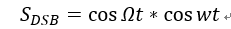若本地相干载波有相位误差ε，则载波信号为：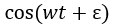，则两者相乘后得到：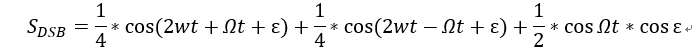经过低通滤波器滤出低频成分：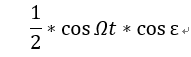可以看到，相位误差对解调信号产生了衰减。
单边带解调
设单频基带信号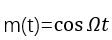，其对载波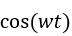进行单边带调制后，取出上边带信号：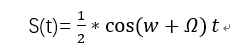若本地相干载波有相位误差ε，则载波信号为：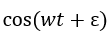，则两者相乘后得到：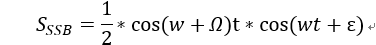化简可以得到：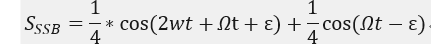经过低通滤波器滤除低频成分：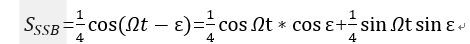第一项是原调制基带信号，但是受到因子cos⁡ε的衰减，第二项是和第一项正交的项，它使接收信号产生失真。


展开全文• 做通信实验时的必备指导书，包括实验一 信号源实验 实验二 信道与眼图实验 实验四 常规双边带调幅与解调实验（AM）实验七 频分复用实验（FDM）等几个通信必做实验，相信能够对您的学习有所助益！
• 信号AM调制解调MATLAB仿真的资料-AM信号相干解调与非相干解调比较.pdf 双边带幅度调制及其MATLAB仿真 幅度调制信号的特性分析及其MATLAB仿真研究 AM信号相干解调与非相干解调比较matlab
• matlab开发-DSB语音信号调制解调双边带调制解调仿真
• 在这里我将用到的是滤波器法，其原理：产生SSB信号的最直观的方法是让双边带信号通过一个边带滤波起保留所需要的一个边带，滤除不要的边带，这只要让它通过一个低通滤波器，而这是很难实现，为此在工程中往往采用...
• 实际相干解调是接收端要恢复出一个与调制载波严格同步的相干载波,相干解调用于对载波被抑止的双边带或单边带信号进行解调。外加载波信号电压加入周步检波器可以有两种方式：一种是将它与接收信号在检波器中相乘，经...
•MATLAB
• 武汉理工大学基于MATLAB的信号调制与解调课程...信号的常规幅度调制单边带幅度调制以及双边带调制 要求完成的主要任务: 包括课程设计工作量及其技术要求以及说明书撰写等具体要求 采用MATLAB选用适当的函数或矩阵进行如
• 通信电子线路中的部分电路仿真，包括集电极调幅电路和双边带信号的解调电路
• 模拟调制，幅度、相位、频率的调制与解调. 调幅波/抑制载波双边带调幅/残留边带调幅/角度调制和解调
• 文章目录一、模拟调制1 幅度调制1.1标准调幅1.2抑制载波双边带调幅1.3单边带调幅1.4残留边带调幅1.5幅度调制的解调2角度调制2.1调频2.2调相2.3解调 一、模拟调制 1 幅度调制 1.1标准调幅 1.2抑制载波双边带调幅 1.3...matlab
• ## I/Q 解调原理

千次阅读 2018-12-24 23:12:28
IQ 解调器的工作原理可通过将其 RF 输入信号 sRF(t) 表示为两个双边带调制正交载波的组合来说明：  (1)     如图 A 所示，同相分量 I(t) 和正交分量 Q(t) 为基带信号，可被视作一个产生 sRF(t) ...
• （1）普通调幅波（AM）的同步解调分析 （2）抑制载波的双边带调幅波的同步解调
• BPSK信号是抑制载波的双边带信号，信号中不存在载频分量，因而BPSK信号的相干解调需要通过非线性变换，如Costas环、平方环电路、数字锁相环（DPLL）技术等方法提取载频信号，需要较为复杂的载波恢复电路。...
•          如果在AM调制模型中将直流A0去掉，即可得到一种高调制效率的调制方式——抑制载波双边带信号（DSB—SC），简称双边带调制信号（DSB）。其时域表达式为 sDSB(t)=m(t)coswct s_{DSB}(t) = m(t)cosw...
• 系统模型2.1 模拟调制系统的基本系统模型2.2 常规调幅（AM）2.3 抑制载波双边带调幅（DSB-SC）2.4 单边带调幅（SSB）3.抗噪声性能理论分析3.1 常规调幅（AM）3.2 抑制载波双边带调幅（DSB-SC）3.3 单边带调幅（SSB）...
• 这是我们课程设计要求做的MATLAB通信系统仿真----模拟信号的频率调制与解调双边带幅度调制与解调，希望对搞通信仿真的初学者有用！
• 信号AM调制解调MATLAB仿真的资料-幅度调制信号的特性分析及其MATLAB仿真研究.pdf 双边带幅度调制及其MATLAB仿真 幅度调制信号的特性分析及其MATLAB仿真研究 AM信号相干解调与非相干解调比较matlab